Description of Systems

# Description of Systems - Notes | Study Signals and Systems - Electrical Engineering (EE)

 1 Crore+ students have signed up on EduRev. Have you?

Description of Systems

What is a system?

A signal was defined as a mapping from a set of the independent variable (domain) to the set of the dependent variable (co-domain). A system is also a mapping, but across signals, or across mappings . That is, the domain set and the co-domain set for a system are both sets of signals, and corresponding to each signal in the domain set, there exists a unique signal in the co-domain set.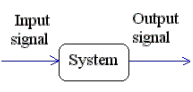In signals and systems terminology, we say; corresponding to every possible input signal, a system “produces” an output signal.

In that sense, realize that a system, as a mapping is one step hierarchically higher than a signal. While the correspondence for a signal is from one element of one set to a unique element of another, the correspondence for a system is from one whole mapping from a set of mappings to a unique mapping in another set of mappings!

Examples of systems

Examples of systems are all around us. The speakers that go with your computer can be looked at as systems whose input is voltage pulses from the CPU and output is music (audio signal). A spring may be looked as a system with the input , say, the longitudinal force on it as a function of time, and output signal being its elongation as a function of time. The independent variable for the input and output signal of a system need not even be the same.

Example of CRO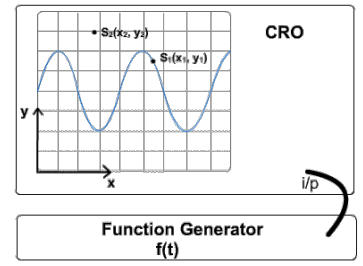An input voltage signal f(t) is provided to the CRO by using a function generator. The CRO (the system) transforms this input function into an image that is displayed on the CRO screen. The luminosity of every point on this display (i.e.value of the signal) is dependent on the x and y coordinates.So, the output S(x, y) has it's independent variable as space, whereas the input independent variable is time.

In fact, it is even possible for the input signal to be continuous-time and the output signal to be discrete-time or vice-versa. For example, our speech is a continuous-time signal, while a digital recording of it is a discrete-time signal! The system that converts any one to the other is an example of this class of systems.

As these examples may have made evident, we look at many physical objects/devices as systems, by identifying some variation associated with them as the input signal and some other variation associated with them as the output signal (the relationship between these, that essentially defines the system depends on the laws or rules that govern the system) . Thus a capacitance with voltage (as a function of time) considered as the input signal and current considered as the output signal is not the same system as a capacitance with, say charge considered as the input signal and voltage considered as the output signal. Why?

The mappings that define the system are different in these two cases.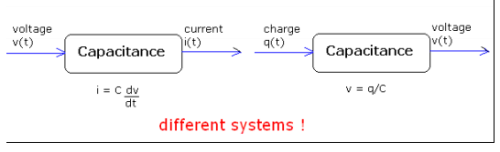We shall next discuss what system description means.

System description

The system description specifies the transformation of the input signal to the output signal. In certain cases, a system has a closed form description. E.g. the continuous-time system with description y(t) = x(t) + x(t-1); where x(t) is the input signal and y(t) is the output signal. Not all systems have such a closed form description. Just as certain "pathological" functions can only be specified by tabulating the value of the dependent variable against all values of the independent variable; some systems can only be described by tabulating the output signal against all possible input signals.

Explicit and Implicit Description

When a closed form system description is provided, it may either be classified as an explicit description or an implicit one.

For an explicit description, it is possible to express the output at a point, purely in terms of the input signal. Hence, when the input is known, it is easily possible to find the output of the system, when the system description is Explicit. In case of an Explicit description, it is clear to see the relationship between the input and the output. e.g. y(t) = { x(t) }2 + x(t-5).

In case the system has an Implicit description, it is harder to see the input-output relationship. An example of an Implicit description is y(t) - y(t-1) x(t) = 1. So when the input is provided, we are not directly able to calculate the output at that instant (since, the output at 't-1' also needs to be known). Although in this case also, there are methods to obtain the output based solely on the input, or, to convert this implicit description into an explicit one. The description by itself however is in the implicit form.

The mapping involved in systems

We shall next discuss the idea of mapping in a system in a little more depth.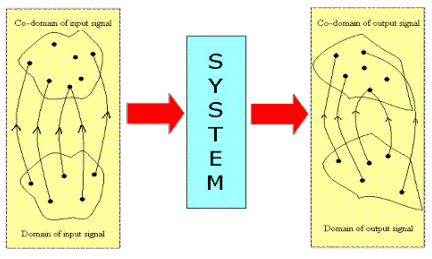A signal maps an element in one set to an element in another. A system, on the other hand maps a whole signal in one set to a signal in another. That is why a system is called a mapping over mappings. Therefore, the value of the output signal at any instant of time (remember "time" is merely symbolic) in general depends on the whole input signal. Thus, even if the independent variable for the input and output signal are the same (say time t), do not assume the value the output signal at, say t = 5

depends on only the value of the input signal at t = 5. For example, consider the system with description: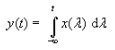The output at, say t = 5 depends on the values of the input signal for all t <= 5.

Henceforth; we shall call systems with both input and output signal being continuous-time as continuous-time systems , and those with both input and output signal being discrete-time as discrete-time systems. Those that do not fall into either of these classes (i.e. input discrete-time and output continuous-time and vice-versa) we shall call hybrid systems. Now that the necessary introductions are done, we can get on to system properties.

The document Description of Systems - Notes | Study Signals and Systems - Electrical Engineering (EE) is a part of the Electrical Engineering (EE) Course Signals and Systems.
All you need of Electrical Engineering (EE) at this link: Electrical Engineering (EE)

## Signals and Systems

40 videos|44 docs|33 tests
 Use Code STAYHOME200 and get INR 200 additional OFF

## Signals and Systems

40 videos|44 docs|33 tests

Track your progress, build streaks, highlight & save important lessons and more!

,

,

,

,

,

,

,

,

,

,

,

,

,

,

,

,

,

,

,

,

,

;